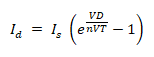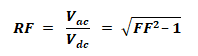# Diode Formulas & Equations – Zenner, Schockley & Rectifier

## Schockley, Zenner & Diode Rectifier Formulas and Equations

### Diode Equation for I-V Curve

The I-V curve (diode characteristic curve) can be find by the following no linear equations. This equation is also known as Ideal Equation of Diode or Diode Law.

i = IS ​( eqv/kT – 1 )

Where:

• i = Current flowing through the diode
• Is = Reverse or dark saturation current (Typical value for silicon is 10-12 Amperes)
• e = Base of the neutral logarithm (2.71828)
• q = Charge on electron (1.602 x 10-19) in coulombs (Absolute Value of electron charge).
• v = Applied voltage across the diode
• k = Boltzmann’s constant (1.380 x 10-23 jouals/Kelvin)
• T = Absolute Temperature in Kelvin (Typical Room Temp is 300 Kelvin)### Schockley Diode Equation:Where

• I= current through the diode
• V= diode voltage
• Is = leakage or reverse saturation current
• n = emission coefficient or ideality factor, for germanium n=1, for silicon it ranges in 1.1-1.8.
• V= thermal voltage which isWhere

• q = charge of electron = 1.6022 x 10-19 coulomb
• T = absolute temperature in Kelvin (K = 273 + °C)
• k = Boltzmann’s constant = 1.3806 x 1023 J/K

### Zenner Diode Formulas & Equations

You may check the Zener diode based regulator calculator in the previous post.

Series Current

IS = VIN – VZ/RS    …..    (Ohm’s Law)

Zener Current

IZ = IS – IL

IL = VL/RL

VVZ

∆VL = IZ RZ

Output (Regulated) Voltage

• VOut = VIN – I R
• VOut = VIN – (IZ + IL)/RS
• VOut = (VIN – IS)/RS

Series Resistance

RS = (V– Vout) / (IZ + IL) = (V– Vout)/(IS)

Max Series Resistance

RS (MAX) = RL (MIN) x [(VIN (MIN) / VZ) -1]

RS (MAX) = RL (MIN) x [(VIN (MIN) – VZ)/IL(MAX)]

Value of Resistor

R = [(VIN (MIN) – VOUT)/(IL + 10)]

Power of Resistor

RP = (VIN (Max) – Vout) 2­­ / R

Power of Zener Diode

ZP = (VIN(MIN) – VOUT) / R) x VOUT

Output Ripple

VR (OUT) ≈ VR (IN) x (RZ/RS)

### Diode Rectifier Equations:

A rectifier’s output contains DC as well as AC components, So;

#### Output DC Power:

Pdc = Vdc Idc­

Where

• Vdc is the average output voltage
• Idc­ is the average output current

#### Output AC Power:

Pac = Vrms Irms

Where

• Vrms Is the rms of output voltage
• Irms is the rms of output current

#### Rectifier Efficiency:

The efficiency of the rectifier denote by η is given by:Where

• Pdc is the output DC power
• Pac is the output AC power

#### Output AC Voltage:

The rms of AC component of the output voltage is:#### Form Factor:

The ratio of RMS voltage to the average dc voltage,#### Ripple Factor:

It’s the ratio between the AC and DC component of the rectifier. It shows the purity of the DC output.Related Formulas and Equations Posts: